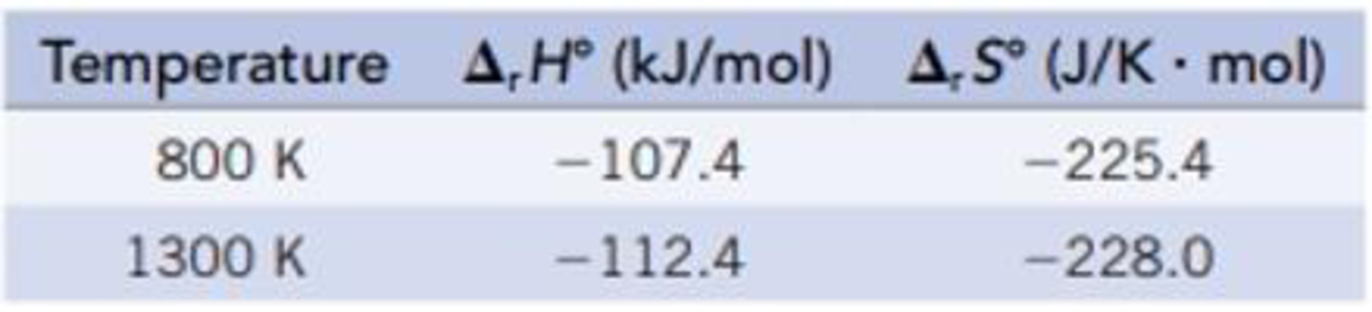Chapter 18, Problem 105SCQ

Chapter
Section
Textbook Problem

The Haber-Bosch process for the production of ammonia is one of the key industrial processes in developed countries.N2(g) + 3H2(g) ⇄ 2NH3(g)(a) Calculate ΔrG° for the reaction at 298 K, 800 K, and 1300 K. Data at 298 K are given in Appendix L Data for the other temperatures are as follows:How does the ΔrG° change with temperature?(b) Calculate the equilibrium constant for the reaction at 298 K, 800 K, and 1300 K.(c) At what temperature (298 K, 800 K, or 1300 K) is the mole fraction of NH3 the greatest?

(a)

Interpretation Introduction

Interpretation:

The free energy change values for given temperatures and the response of it with respect to the temperature should be identified.

Concept introduction:

The Gibbs free energy or the free energy change is a thermodynamic quantity represented by ΔrG. It can be calculated in a similar manner as entropy and enthalpy.  The expression for the free energy change is:

ΔrG°fG°(products)fG°(reactants)

ΔGo is related to the equilibrium constant K by the equation,

ΔrGo= -RTlnKp

The rearranged expression is,

Kp=e-ΔrGoRT

It is also related to entropy and entropy by the following expression,

ΔGo= ΔHo-TΔSo

Here, ΔHo is the change in enthalpy and ΔSo is the change in entropy.

Explanation

The ΔrGo for the given reaction at different temperature is calculated below.

Given:

Refer to Appendix L for the values of standard free energy, standard entropy and standard enthalpy values.

The given reaction is,

N2(g)+3H2(g)2NH3(g)

At 298 K,

The ΔfG° for NH3(g) is 16.37 kJ/mol.

The ΔfG° for H2(g) is 19.33 kJ/mol.

The ΔfG° for N2(g) is 0 kJ/mol.

ΔrG°fG°(products)fG°(reactants)=[(2 mol NH3(g)/mol-rxn)ΔfG°[NH3(g)]-[(3 mol H2(g)/mol-rxn)ΔfG°[H2(g)]+(1 mol N2(g)/mol-rxn)ΔfG°[N2(g)]]]

Substitute the values,

ΔrG°=[(2 mol NH3(g)/mol-rxn)(-16

(b)

Interpretation Introduction

Interpretation:

The equilibrium constant values for given temperatures should be identified.

Concept introduction:

The Gibbs free energy or the free energy change is a thermodynamic quantity represented by ΔrG. It can be calculated in a similar manner as entropy and enthalpy.  The expression for the free energy change is:

ΔrG°fG°(products)fG°(reactants)

ΔGo is related to the equilibrium constant K by the equation,

ΔrGo= -RTlnKp

The rearranged expression is,

Kp=e-ΔrGoRT

It is also related to entropy and entropy by the following expression,

ΔGo= ΔHo-TΔSo

Here, ΔHo is the change in enthalpy and ΔSo is the change in entropy.

(c)

Interpretation Introduction

Interpretation:

The temperature at which mole fraction of given species will be greatest should be identified.

Concept introduction:

The Gibbs free energy or the free energy change is a thermodynamic quantity represented by ΔrGo. It can be calculated in a similar manner as entropy and enthalpy.  The expression for the free energy change is:

ΔrG°fG°(products)fG°(reactants)

ΔGo is related to the equilibrium constant K by the equation,

ΔrGo= -RTlnKp

The rearranged expression is,

Kp=e-ΔrGoRT

It is also related to entropy and entropy by the following expression,

ΔGo= ΔHo-TΔSo

Here, ΔHo is the change in enthalpy and ΔSo is the change in entropy.

Still sussing out bartleby?

Check out a sample textbook solution.

See a sample solution

The Solution to Your Study Problems

Bartleby provides explanations to thousands of textbook problems written by our experts, many with advanced degrees!

Get Started

Calculate your protein RDA.

Understanding Nutrition (MindTap Course List)

What impact has recombinant DNA technology had on genetics and society?

Human Heredity: Principles and Issues (MindTap Course List)

Compare the chemical behavior of benzene and cyclohexene.

Chemistry for Today: General, Organic, and Biochemistry